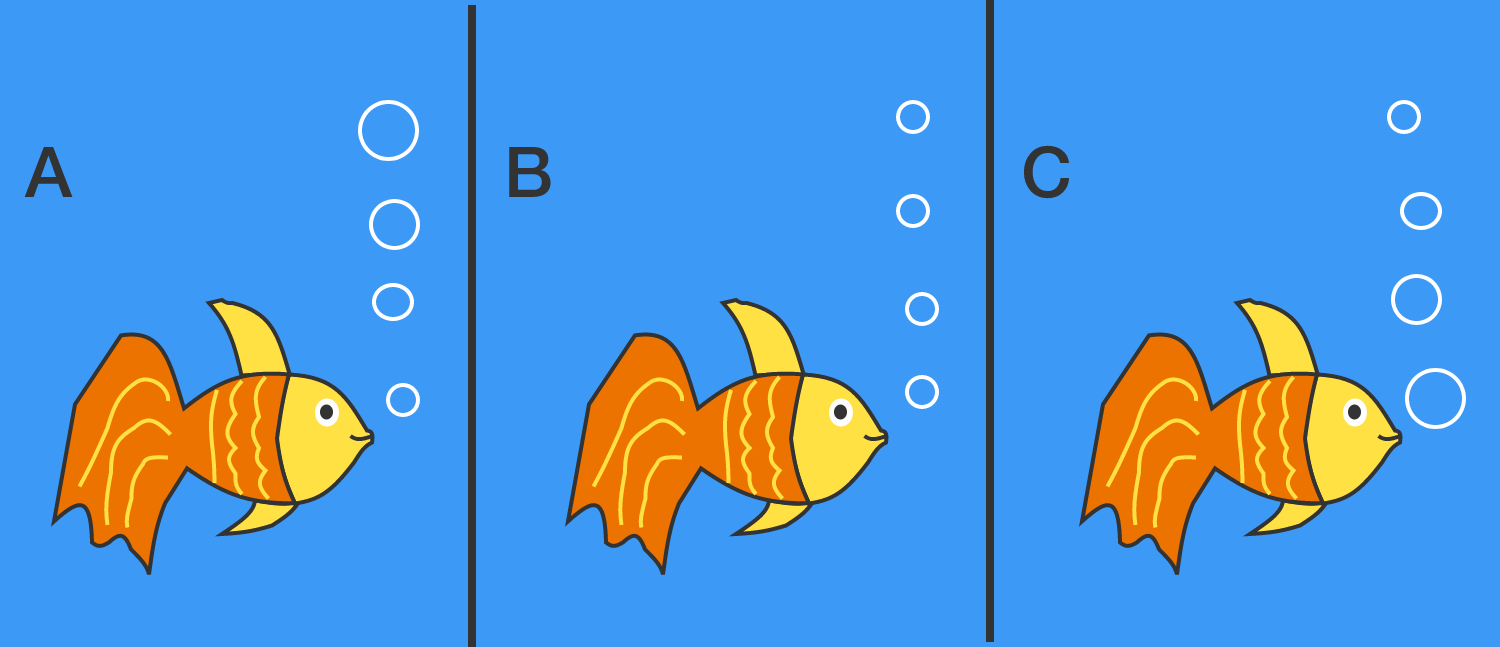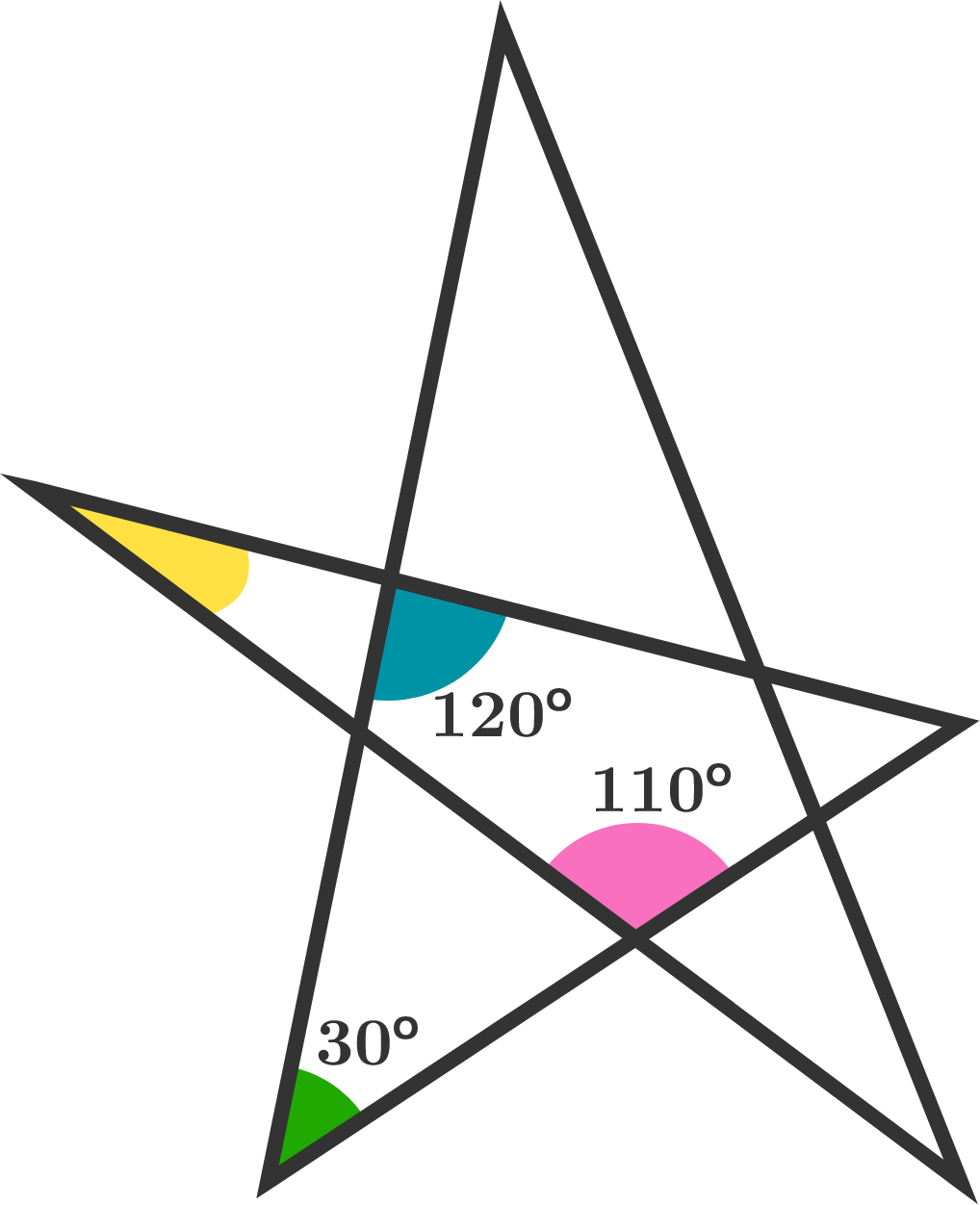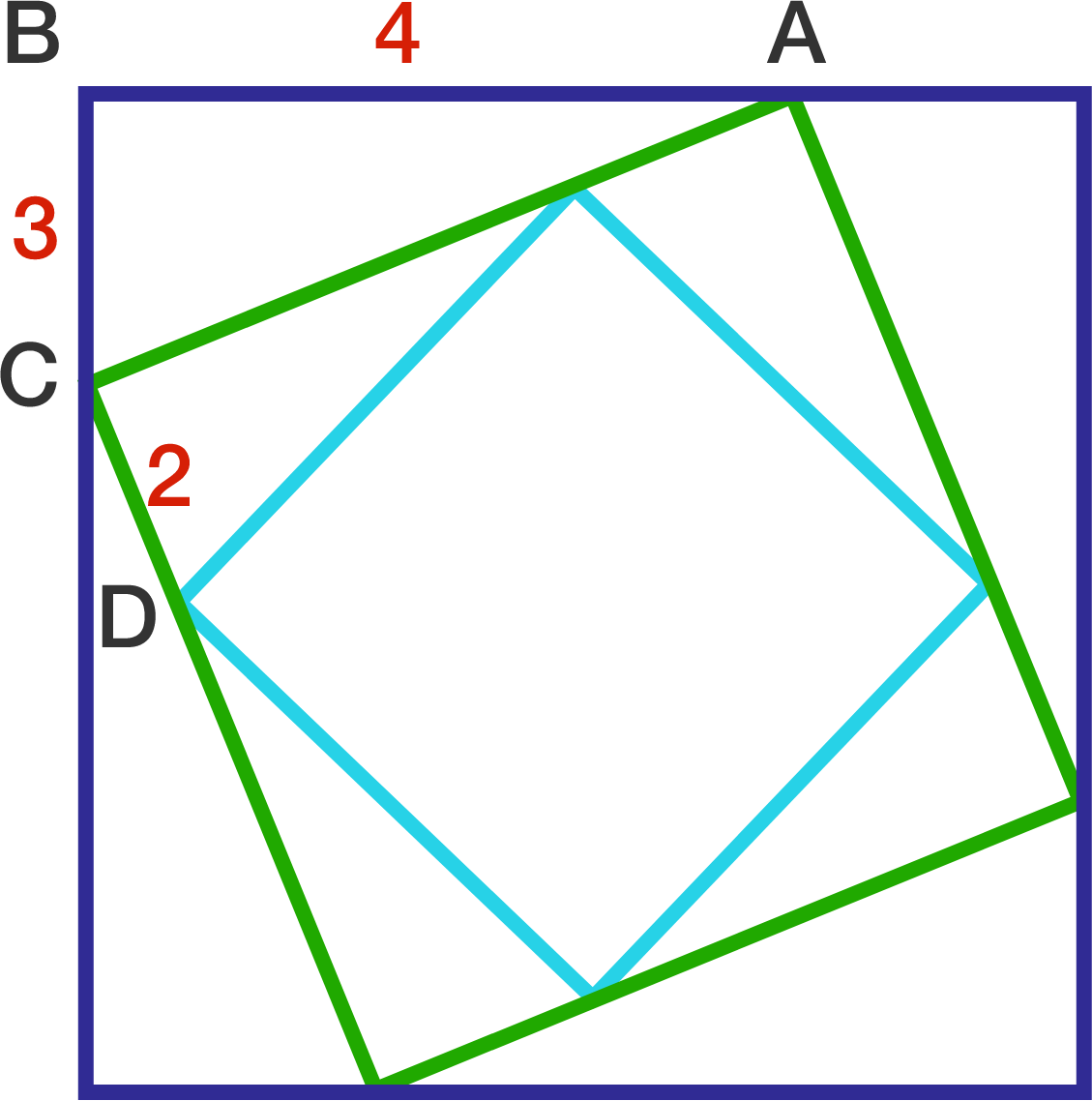# Problems of the Week

Contribute a problem

# 2017-03-27 Basic

$\begin{array} { l l l l } & A & A \\ & A & A \\ & & A \\ & & A \\ + & & A \\ \hline 1 & 0 & 0 \end{array}$

What is $A$?When a goldfish puffs out an equal amount of air each time he mumbles under water, how do the bubbles form?

A. The bubbles gradually get larger as they get closer to the surface.
B. The bubbles always stay the same size throughout the ascent.
C. The bubbles gradually shrink as they reach the surface.
D. None of the above. The bubbles will randomly vary in size.

What are all the possible perimeters of an isosceles triangle with sides of lengths 5 and 16?In the star, what is the yellow angle?The diagram consists of three nested squares. The lengths of the line segments are $AB = 4, BC = 3,$ and $CD = 2$.

What is the area of the smallest square?

×